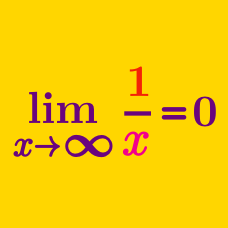Calculus

# Limits of Functions: Level 4 Challenges

$\large \lim_{x \to a} \bigg( 2 - \frac{a}{x} \bigg)^{\tan \frac{\pi x}{2a}} = e^{n}$

Find approximate value of $$n$$.

$\large \displaystyle \lim_{n \to \infty} \left(\dfrac{a+\sqrt[n]{b}-1}{a}\right)^{n}$

Calculate the limit above in terms of $$a$$ and $$b$$, where $$a$$ and $$b$$ are constants with $$b\geq0$$.

Let $$f:(1,\infty) \rightarrow (0,\infty)$$ be a continuous decreasing function with $\large \lim_{x\to\infty} \dfrac{f(4x)}{f(8x)} = \, 1$

Then

$\large \lim_{x\to\infty} \dfrac{f(6x)}{f(8x)} = \, ?$

Let $$\{x_n\}$$ be a sequence such that $$x_1=1,\ x_nx_{n+1}=2n$$ for $$n\ge 1$$.

Find $$\displaystyle \lim_{n\to\infty} \dfrac{|x_{n+1}-x_n|}{\sqrt{n}}.$$

Compute $\large \lim_{ n\to{\infty}}{\dfrac{ 2016(1^{2015}+2^{2015}+ \cdots +n^{2015}) - n^{2016}}{2016(1^{2014}+2^{2014}+\cdots+n^{2014})}}.$

×